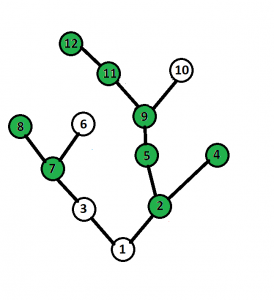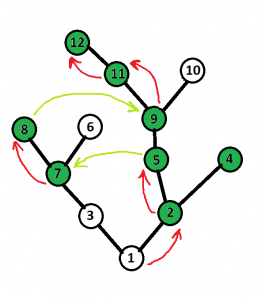Related Articles

# Traversal of tree with k jumps allowed between nodes of same height

• Difficulty Level : Expert
• Last Updated : 20 May, 2019

There is a tree with N nodes and node 1 is the root node. Each node of the tree can contain fruit or not.
Initially, you are at the root node and start climbing on the tree.
You can jump from a node to any node at the same level(i.e. the height of nodes from the root are same),
During climbing from root node you can only make maximum K jumps. (K < 20)
Now you have to climb on the tree (from root node-> any leaf node) in such a way so that you can collect maximum no of fruits.
Example :

```Input Tree :
Number of Nodes N = 12
Number of jumps allowed : 2
Edges:
1 2
1 3
2 4
2 5
5 9
9 10
9 11
11 12
3 7
7 6
7 8
no of node having fruit(nf) : 8
Nodes Containing Fruits(lvn) : 2 4 5 7 8 9 11 12
Output: 7
```

Tree for above testcase :Explanation:## Recommended: Please try your approach on {IDE} first, before moving on to the solution.

Approach: The idea is to use DFS to create a Height Adjacency List of the Nodes and to store the parents. Then use another dfs to compute the maximum no of special nodes that can be reached using the following dp state:

```dp[current_node][j] = max( max{ dp[child_i][j], for all children of current_node },
max{ dp[node_at_same_height_i][j - 1],
for all nodes at same height as current_node} )
```

Thus, dp[Root_Node][Total_no_of_Jumps] gives the answer to the problem.

Below is the implementation of above approach :

 `// Program to demonstrate tree traversal with ``// ability to jump between nodes of same height ``#include  ``using` `namespace` `std; ``   ` `#define N 1000 ``   ` `vector<``int``> H[N]; ``   ` `// Arrays declaration ``int` `Fruit[N]; ``int` `Parent[N]; ``int` `dp[N]; ``   ` `// Function for DFS ``void` `dfs1(vector<``int``> tree[], ``int` `s, ``          ``int` `p, ``int` `h) ``{ ``    ``Parent[s] = p; ``    ``int` `i; ``    ``H[h].push_back(s); ``    ``for` `(i = 0; i < tree[s].size(); i++) { ``        ``int` `v = tree[s][i]; ``        ``if` `(v != p) ``            ``dfs1(tree, v, s, h + 1); ``    ``} ``} ``   ` `// Function for DFS ``int` `dfs2(vector<``int``> tree[], ``int` `s, ``         ``int` `p, ``int` `h, ``int` `j) ``{ ``    ``int` `i; ``    ``int` `ans = 0; ``    ``if` `(dp[s][j] != -1) ``        ``return` `dp[s][j]; ``   ` `    ``// jump ``    ``if` `(j > 0) { ``        ``for` `(i = 0; i < H[h].size(); i++) { ``            ``int` `v = H[h][i]; ``            ``if` `(v != s) ``                ``ans = max(ans, dfs2(tree, v, ``                        ``Parent[v], h, j - 1)); ``        ``} ``    ``} ``   ` `    ``// climb ``    ``for` `(i = 0; i < tree[s].size(); i++) { ``        ``int` `v = tree[s][i]; ``        ``if` `(v != p) ``            ``ans = max(ans, dfs2(tree, v, s, h + 1, j)); ``    ``} ``   ` `    ``if` `(Fruit[s] == 1) ``        ``ans++; ``    ``dp[s][j] = ans; ``   ` `    ``return` `ans; ``} ``   ` `// Function to calculate and ``// return maximum number of fruits ``int` `maxFruit(vector<``int``> tree[], ``             ``int` `NodesWithFruits[], ``             ``int` `n, ``int` `m, ``int` `k) ``{ ``    ``// reseting dp table and Fruit array ``    ``for` `(``int` `i = 0; i < N; i++) { ``        ``for` `(``int` `j = 0; j < 20; j++) ``            ``dp[i][j] = -1; ``        ``Fruit[i] = 0; ``    ``} ``   ` `    ``// This array is used to mark ``    ``// which nodes contain Fruits ``    ``for` `(``int` `i = 0; i < m; i++) ``        ``Fruit[NodesWithFruits[i]] = 1; ``   ` `    ``dfs1(tree, 1, 0, 0); ``    ``int` `ans = dfs2(tree, 1, 0, 0, k); ``   ` `    ``return` `ans; ``} ``   ` `// Function to add Edge ``void` `addEdge(vector<``int``> tree[], ``int` `u, ``int` `v) ``{ ``    ``tree[u].push_back(v); ``    ``tree[v].push_back(u); ``} ``   ` `// Driver Code ``int` `main() ``{ ``    ``int` `n = 12; ``// Number of nodes ``    ``int` `k = 2; ``// Number of allowed jumps ``   ` `    ``vector<``int``> tree[N]; ``   ` `    ``// Edges ``    ``addEdge(tree, 1, 2); ``    ``addEdge(tree, 1, 3); ``    ``addEdge(tree, 2, 4); ``    ``addEdge(tree, 2, 5); ``    ``addEdge(tree, 5, 9); ``    ``addEdge(tree, 9, 10); ``    ``addEdge(tree, 9, 11); ``    ``addEdge(tree, 11, 12); ``    ``addEdge(tree, 3, 7); ``    ``addEdge(tree, 7, 6); ``    ``addEdge(tree, 7, 8); ``   ` `    ``int` `NodesWithFruits[] = { 2, 4, 5, 7, 8, 9, 11, 12 }; ``   ` `    ``// Number of nodes with fruits ``    ``int` `m = ``sizeof``(NodesWithFruits) / ``sizeof``(NodesWithFruits); ``   ` `    ``int` `ans = maxFruit(tree, NodesWithFruits, n, m, k); ``   ` `    ``cout << ans << endl; ``   ` `    ``return` `0; ``} `
Output:
```7
```

Time Complexity : O(n*n*k) (worst case, eg: 2 level tree with the root having n-1 child nodes)My Personal Notes arrow_drop_up